#The Model Student: A Primal Skyline (Part 3)

Overload Journal #94 - December 2009 + Programming Topics   Author: Richard Harris
The prime factors of the integers show some repeating patterns. Richard Harris investigates whether they have fractal properties.

In part 1 of this investigation I introduced some of the great questions, both answered and unanswered, about those elitists of the integers, the primes; those whole numbers greater than or equal to 2 that will not suffer to be wholly divided by any other than themselves and the singularly noble 1.

We took a look at Euclid's impressively straightforward proof of the infinitude of the primes and introduced the prime number theorem which states that the number of primes less than or equal to a given number n, denoted by the function p, is approximately equal towhere the lim term denotes the limit of the fraction as n grows larger and larger.

Moving on, we considered the factorisations of the integers; the unique sets of primes that when multiplied together result in any given integer greater than 0. I pointed out that 1 can be considered the product of no primes and that 0 can be considered the product of negative infinity of them, or equivalently of dividing 1 by infinitely many primes.

We then took a look at a relative of p, the function that counts the number of prime factors of its argument, Ω. For example, the number 42 can be represented as the product of the primes 2 × 3 × 7 and hence Ω(42) = 3.

Recall that Ω counts repeated prime factors, so that 84, which has the factorisation 22× 3 × 7 has a value of Ω equal to Ω(84) =4.

Building upon this, and in pursuit of a pattern in the factorisations of the integers, I introduced the function ף‎n, defined for non-negative integer n, real number arguments greater than or equal to 0 and less than or equal to 1 and the expression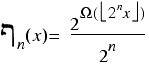Figure 1 illustrates two example graphs of ף‎n‎5 and ף‎7).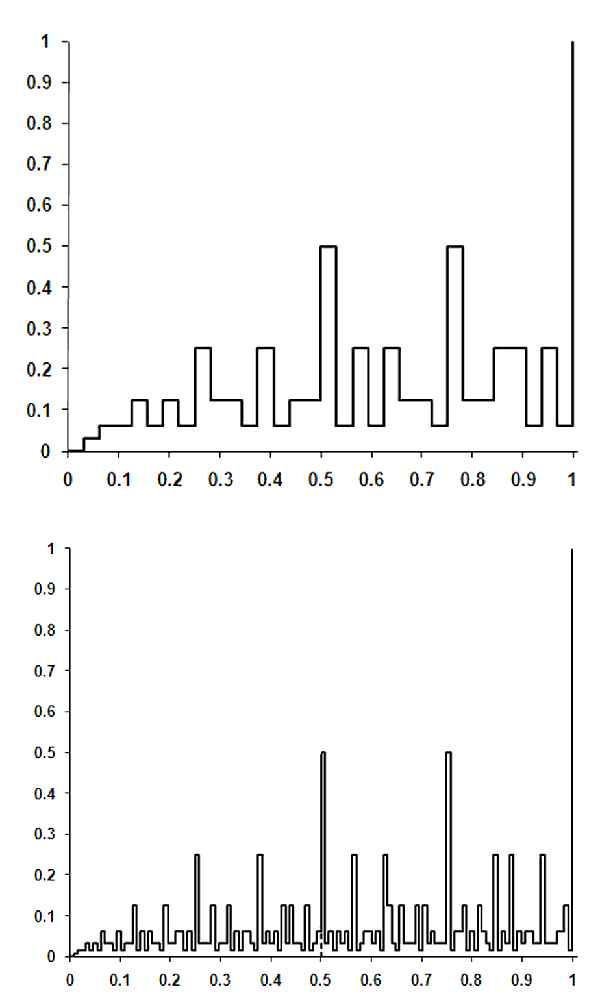Figure 1

We next showed that, for any n greater than 0, ף‎n and ףn+1 are coincident for half the points in the range 0 to 1 and also that the infinite limit, ף, can be entirely recovered from an arbitrarily small range of arguments, both of which bear a resemblance to the properties of many fractals.

In part 2, we gave fractals the sound definition of being objects that have a fractional dimension. Of the many definitions of dimension we chose the Minkowski, or box-counting dimension, defined in terms of the number of rulers of length ε required to cover a curve, N(ε), by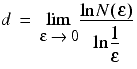where the lim term means the limit of the expression to its right as ε tends to 0.

As a motivating example, I introduced the Koch curve, generated by iteratively replacing every straight in its fundamental element, illustrated in figure 2 (the basic element of the Koch curve), with a scaled down version of itself.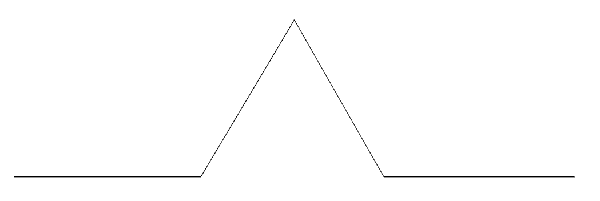Figure 2

A later iteration in the construction of this curve is illustrated in figure 3.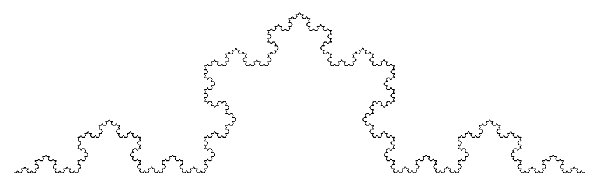Figure 3

Noting that every time we divide the length of the ruler by 3, we increase the number of them required to cover the curve by a factor of 4, we can deduced that the fractal dimension of the Koch curve is approximately 1.2619.

We also demonstrated that the number of rulers of length ε‎n, equal to 1 divided by 2n, required to cover the graph of ף‎n would probably exceed the maximum value of an unsigned long and so implemented an accumulator class to keep track of them, as illustrated in listing 1.

 ``` class accumulator { public: accumulator(); accumulator & operator+=(unsigned long n); operator double() const; private: std::vector n_; }; ``` Listing 1

We reused the count_factors function we implemented in the first part, declared as shown below.

```      template<class FwdIt>
unsigned long
count_factors(unsigned long x, FwdIt first_prime,
FwdIt last_prime);
```

Finally, taking great care to ensure that we couldn't possibly be laid low by integer wrap-around, even for n equal to the number of bits in an unsigned long, we implemented a function to calculate the required number of rulers, whose declaration is given again below:

```      double count_rulers(unsigned long n);
```

## The box-counting dimension of ף∞

You will no doubt be relieved to read that we are at long last ready to investigate whether ף is fractal or not.

The results of the successive approximations of the box-counting dimension calculation using ף‎n for n from 1 to 32 (the number of bits in an unsigned long on my, and I suspect almost everyone's, machine) are given in figure 4.

n d n d n d n d

1

2.0000

9

1.4745

17

1.3612

25

1.2913

2

1.5000

10

1.4575

18

1.3505

26

1.2845

3

1.5283

11

1.4411

19

1.3405

27

1.2781

4

1.5000

12

1.4258

20

1.3311

28

1.2720

5

1.5229

13

1.4114

21

1.3222

29

1.2662

6

1.5181

14

1.3976

22

1.3138

30

1.2606

7

1.5039

15

1.3846

23

1.3059

31

1.2554

8

1.4906

16

1.3725

24

1.2984

32

1.2503

Figure 4

Clearly it has not yet reached its limit and, as illustrated by the graph of these values in figure 5, it's not at all obvious exactly what that limit might be.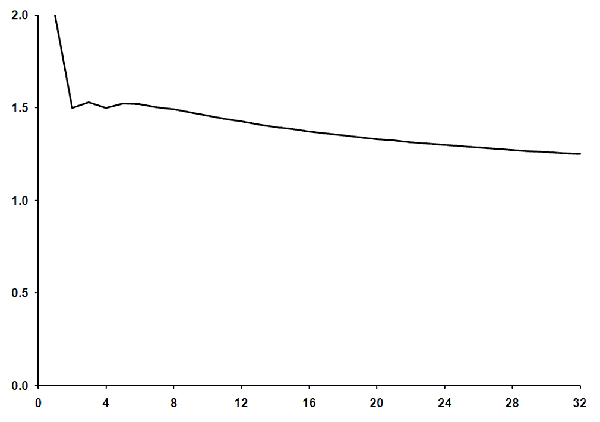Figure 5

That said, since the graph changes fairly smoothly for the larger values of n, we may very well be able to extrapolate it to the limit of n equal to infinity, or equivalently ε‎n equal to 0, and hence determine the fractal dimension of ף.

## The length of ף∞

Rather than consider the successive approximations to the box-counting dimension of ף, hereafter denoted by D‎n, we shall instead consider the lengths of ף‎n for each n, given by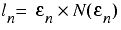Note that the two values are related by the equationsPlotting the length of ף‎n against n, we discover a suspiciously familiar graph, as illustrated in figure 6.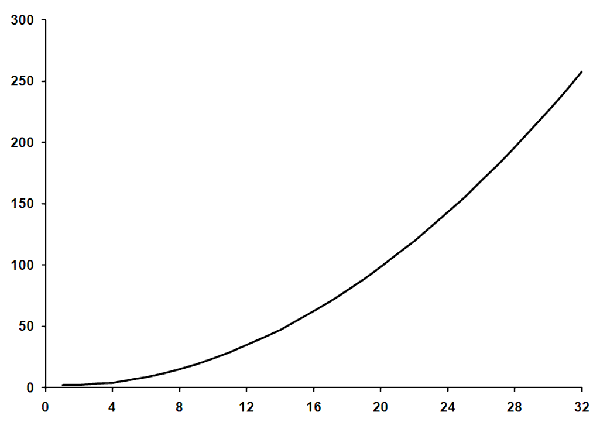Figure 6

In fact, this curve is reasonably well approximated by the formula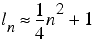and is very well approximated by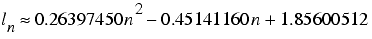especially so for large n.

This implies that the approximate fractal dimension Dף‎n is itself accurately approximated by 1, as shown in derivation 1.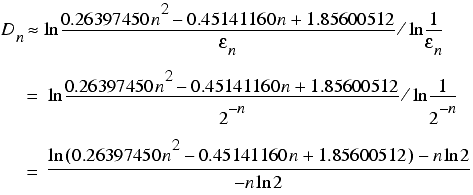As n grows ever larger, so the linear and constant terms in the argument of the first logarithm becomes ever less significant, implying that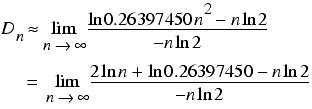and since, for n greater than 1, ln n is always absolutely smaller than n, and significantly so for large n, we have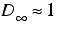Derivation 1

## So it's

Well, whilst I haven't provided anything remotely close to a proof, I very much suspect that it isn't.

Nevertheless, the fact that its length is a function of the length of the ruler used to measure it is still an interesting property. Specifically, since n can be recovered from ε‎n, we have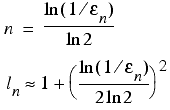The length of a straight line is constant no matter what the length of the ruler, and is in some sense less fractal-like than ף.

Since a fractal has a dimension greater than 1, let's say d, it has a length that is an exponential function of the logarithm of the reciprocal of the length of the ruler, as shown in derivation 2, and is in the same sense more fractal-like than ף.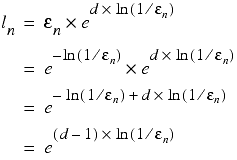Derivation 2

## And what of simple curves?

Recall that we derived the fractal dimension of the circumference of the unit circle using inscribed polygons as illustrated in figure 7.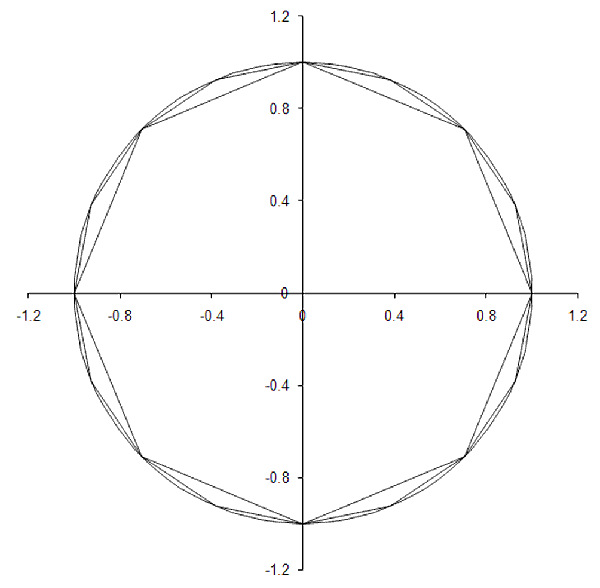Figure 7

Now as our ruler gets smaller and smaller, the measured length of the circumference gets closer and closer to 2π. Any formula relating the log of the length of the ruler to the measured length of the circumference must be dominated by the constant term of 2π, since the log of the ruler length grows increasingly negative as the ruler length decreases. A rough mathematical treatment of this is given in derivation 3.

 Note than an n-sided polygon has sides of length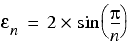Recall how we used Taylor's theorem to approximate a function for small arguments in previous articlesUsing these first 4 terms to approximate the sides of the polygon, we have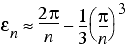The length of the circumference is therefore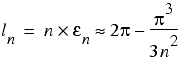Using the first term in our approximation of εn to estimate n in terms of the log of its reciprocal, we have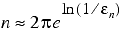and henceDerivation 3

## Mocktals? Or faketals?

What we appear to have found is a curve that sits somewhere between a fractal and a simple curve; a curve whose length increases without limit as the length of the ruler shrinks, but is governed by a polynomial, rather than an exponential, function of the logarithm of the reciprocal of the length of the ruler.

I shall christen these curves mocktals, or perhaps faketals (I can't decide), and use the power of the dominant term in the polynomial function that determines their length, which I shall call the mocktal (or faketal) order or the curve, to compare them to each other.

Note that this order is exactly the sense in which ף is more fractal-like than a simple curve. A simple curve, tending as it does to a constant value, has a mocktal order of 0. A fractal, having a length equal to an exponential function of the logarithm of the reciprocal of the ruler length, has infinite mocktal order. This is because the exponential function can be exactly defined for all arguments, using Taylor's expansion about 0 again, by a polynomial with an infinite number of terms. This is such an important result that the expansion has its own name; the exponential series. The relationship between e, i, π and -1 that we briefly mentioned in the first part of this article can be demonstrated using it, for example.

Of course, thus far we have seen but one example; the mocktal of order 2, ף. Is there a family of such curves, or is our ף a solitary fellow?

## A mocktal sinusoid

Focusing on the partial self similarity exhibited by ף, I propose that we begin our hunt for another mocktal with a function that displays the same property: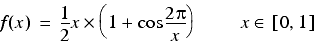Like ף, this passes through both (0,0) and (1,1), as illustrated in figure 8.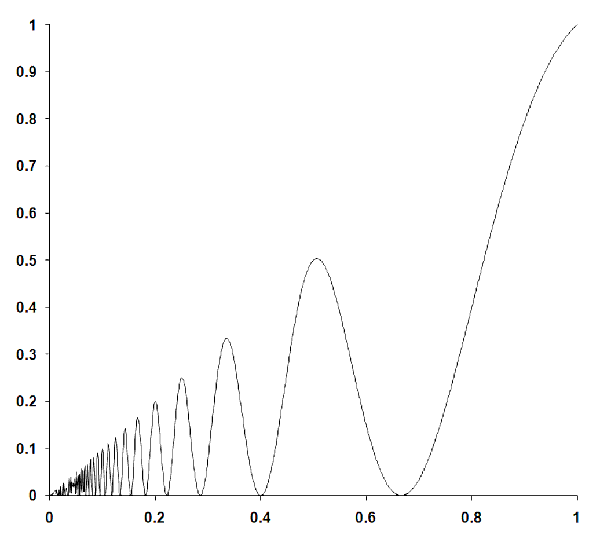Figure 8

Now, unfortunately, this curve isn't so easy to measure with fixed length rulers. We can, however, calculate a reasonable approximation of its length by summing the distances between points on the graph at fixed steps along the x axis. Specifically, with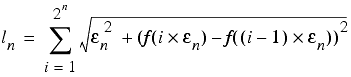where, once again, ε‎n is equal to 1 divided by 2n.

Since we do not have a fixed length ruler in this case, we shall instead use the average distance between the points we use to calculate the length of the curve as an analogue for the ruler length: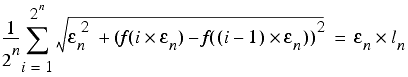Because of this lack of a fixed length ruler, we cannot use our accumulator to count the number of rulers and so shall have to accept the potential precision issues that might arise during the calculation and settle instead for accumulating the length of the curve with a double. Fortunately, the distances between subsequent points in the graph increase as we move from left to right, greatly mitigating our exposure to loss of precision.

The code to calculate the length of this sinusoid is a reasonably straightforward adaptation of the count_rulers function, as illustrated in listing 2.

 ``` double sinusoid_length(unsigned long n) { static const int dig = std::numeric_limits::digits; static const double pi = 2.0*acos(0.0); if(n>dig) throw std::invalid_argument(""); const unsigned long upper_bound = ((n==dig) ? 0UL : (1UL<

Plotting the lengths returned by this function for n from 1 to 32 against the logarithm of the reciprocal of the average distance between the points quite strikingly reveals the mocktal order of this curve, as illustrated in figure 9.Figure 9

As the ruler reaches its smallest values, the graph approaches a straight line with a slope of approximately 1.05. Hence, I believe we can be reasonably confident that this curve has a mocktal order of 1 and consequently falls precisely between a simple curve and ף.

## To be a curve, in the summertime, close to a fractal

The mocktal orders of these two curves are infinitely smaller than that of a true fractal, since fractals have infinite mocktal order, but crucially they are not equal to 0. In this sense, these curves can be thought of as infinitesimally fractal-like.

Infinitesimal numbers were introduced in the original, somewhat hand-waving, definition of the calculus. Defined as numbers smaller than any of the real numbers, but nevertheless greater than 0, their very existence has always been in question. A great deal of effort was expended to define the calculus without them during the 19th century, a time during which the modern, relentlessly rigorous approach to mathematics was adopted.

Those of you who have studied the mathematical subject of analysis will appreciate just how much more convoluted the calculus is without them.

## Non-standard numbers

In more recent times, the infinitesimal numbers have enjoyed something of a resurgence with both the surreal numbers [Knuth74] and the non-standard numbers [Robinson74] providing them with a secure footing.

Just as the real numbers can be represented with an infinite sequence of integers, so the non-standard numbers can be represented with an infinite sequence of reals.

The familiar real numbers are those sequences which, at least after some point in the sequence, have elements forever equal to a given real number.

For example, the non-standard number

`    (1,2,3,π,π,π,π,...)`

is equal to π.

Similarly, we can strictly order the non-standard numbers by defining one to be less than another if, after some point in both their sequences, the elements of the first are always less than the elements of the second. Strictly speaking, given two non-standard numbers a and b defined by

```    a = (a0,a1,a2,a3,a4,...);
b = (b0,b1,b2,b3,b4,...);```

we say that a is less than b if there is some n for which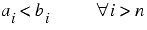where the upside down A means 'for all'.

Finally, we define arithmetic operations on the non-standard numbers by applying the operations element by element to the sequences that represent them. For example, adding a and b yields

`  a+b = (a0+b0,a1+b1,a2+b2,a3+b3,a4+b4,...);`

Given these definitions we can construct non-standard numbers that are greater than 0, but less than any real number. For example, the sequence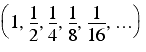consists of elements that are always greater than 0, but growing ever smaller, must after some element always be less than any given real number.

Note that we can also define strictly ordered infinities in the same fashion. For example, inverting the infinitesimal above yields

`    (1,2,4,8,16,...)`

which by our definitions is greater than any real number.

## Mocktals, fractals, infinities and infinitesimals

If we choose a universal sequence of ruler lengths, εף‎n, we can relate straight lines to the non-standard representation of the real numbers since the length of a straight line measured by those rulers is an infinite sequence of identical numbers.

Simple curves, whose length gets closer and closer to some limit as the ruler gets shorter, can be similarly related to non-standard numbers that differ infinitesimally from that limit.

Finally, the mocktals are in this sense equivalent to non-standard infinities ranked by their mocktal order and hence all are smaller than the non-standard infinities to which we can map the fractals.

Dividing any of the mocktal non-standard infinities by any of the fractal non-standard infinites yields an ultimately ever decreasing sequence of numbers which is, by definition, a non-standard infinitesimal.

So there we have it. A mathematically sound mapping by which the mocktals can be described as both infinitesimally fractal-like and yet infinitely more so than simple curves, and an object lesson in the care that must be taken when pushing C++ integers to their very limits to boot.

In parting, I should like to pose the question of whether we can construct curves with any given mocktal order, even non-integer. I haven't found any more yet, but then I haven't really looked very hard.

If you do, dear reader, I would be delighted to hear about them.

## Acknowledgements

With thanks John Paul Barjaktarevic and Lee Jackson for proof reading this article.

## References and further reading

[Devlin05] Devlin, K., The Millenium Problems, Granta Books, 2005.

[Knuth74] Knuth, D., Surreal Numbers, Addison-Wesley, 1974.

[Robinson74] Robinson, A., Non-Standard Analysis, Princeton University Press, 1974.